Main Content

# 5G NR Downlink CSI Reporting

The example shows how to compute the downlink channel state information (CSI) parameters such as the channel quality indicator (CQI) and precoding matrix indicator (PMI) for multiple input multiple output (MIMO) scenarios considering type-1 single panel codebooks, as defined in TS 38.214 Section 5.2.2, over a tapped delay line (TDL) channel.

### Introduction

CSI parameters are the quantities related to the state of a channel that are reported by the user equipment (UE) to the access network node (gNB) as a feedback. The CSI feedback includes several parameters, such as the CQI, the PMI with different codebook sets, and the rank indicator (RI). The channel state information reference signal (CSI-RS) is used to measure the CSI feedback. When the gNB receives the CSI parameters, it can schedule the downlink data transmissions (such as modulation scheme, code rate, number of transmission layers, and MIMO precoding) accordingly. The figure shows the overview of CSI-RS transmission, CSI computation and feedback, and the transmission of downlink data that is scheduled based on the CSI parameters.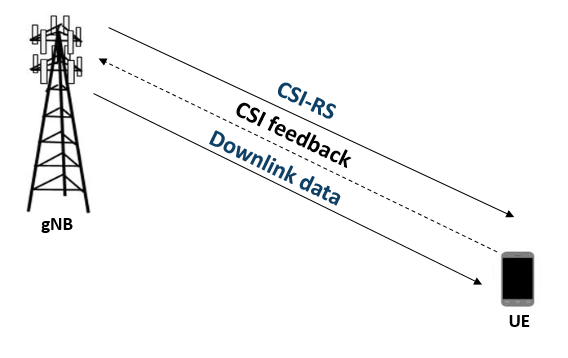#### RI Selection

The RI defines the number of possible transmission layers for the downlink transmission under specific channel conditions. It is also termed as the maximum number of uncorrelated paths that can be used for the downlink transmission. Other CSI parameters like the PMI and CQI are computed based on the rank provided by the RI. The current example does not support RI computation. The CQI and PMI parameters are computed in the example assuming a known rank.

#### PMI Selection

The PMI is a set of indices corresponding to a precoding matrix that can be used for the downlink data transmission. A codebook is selected from a set of possible codebooks, defined in TS 38.214 Tables 5.2.2.2.1-1 to 5.2.2.2.1-12, based on the possible number of transmission layers for the downlink transmission and CSI reporting configuration parameters such as antenna panel dimensions and the codebook mode. The codebook consists of a set of precoding matrices. For given channel conditions, the signal to interference and noise ratio (SINR) is computed at the receiver side by considering all precoding matrices from the selected codebook. The indices of the precoding matrix, which gives the maximum SINR, are reported as the PMI (constituting the two indices set ${\mathit{i}}_{1},{\mathit{i}}_{2}\text{\hspace{0.17em}},$ as defined in TS 38.214 Section 5.2.2.2.1). The figure shows the procedure for PMI selection.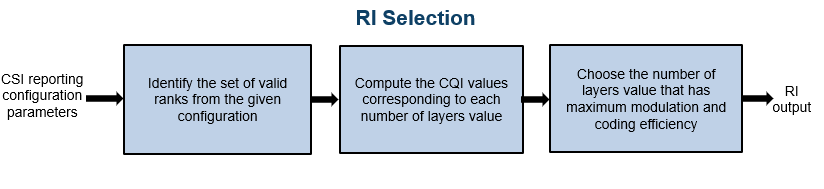#### CQI Selection

The CQI is an indicator of channel quality. The CQI index is a scalar value from 0 to 15. It provides the information about the highest modulation scheme and the code rate (MCS) suitable for the downlink transmission to achieve the required block error rate (BLER) for a given channel conditions. The highest CQI index is selected when a single physical downlink shared channel (PDSCH) transport block with a combination of modulation scheme, target code rate, and transport block size that correspond to the CQI index and that occupy a group of downlink physical resource blocks termed the CSI reference resource, can be received with a transport block error probability not exceeding:

• 0.1 for 'cqi-Table' as 'table1' or 'table2'

• 0.00001 for 'cqi-Table' as 'table3'

The 'cqi-Table' is a higher layer parameter that corresponds to the CQI versus MCS table, and SINR lookup table is computed for this table. This example uses a 0.1 BLER (corresponding to a 90 percent throughput) and 'cqi-Table' as 'table1' (TS 38.214 Table 5.2.2.1-2). The relationship between the CQI indices, the modulation scheme, and the code rate (from which the transport block size is derived) is described in TS 38.214 Tables 5.2.2.1-2 to 5.2.2.1-4. The CSI reference resource is defined in TS 38.214 Section 5.2.2.5.

The CQI value is computed by considering the SINR values that correspond to the reported PMI, using the precalculated lookup table of the CQI index versus ${\mathrm{SINR}}_{\mathit{i}}$, as a reference. The SINR values across all layers (corresponding to the reported PMI) are mapped to each codeword. For each codeword, the respective SINR is compared with the ${\mathrm{SINR}}_{\mathit{i}}$ values from the table and then, the CQI value against the maximum SINR, which is less than the codeword SINR is selected. The CQI value is selected such that the BLER is less than or equal to 0.1 when operated at ${\mathrm{SINR}}_{\mathit{i}}$. If a CQI index of 1 does not satisfy the BLER condition, then the CQI index is considered as 0. The figure shows the procedure for CQI selection.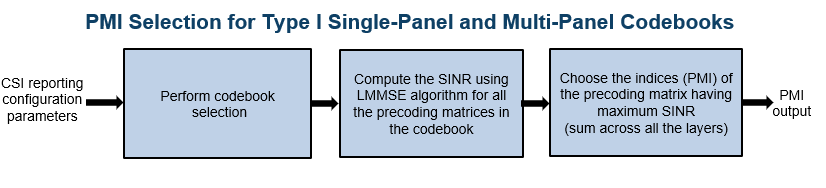#### CQI Versus SINR Table

The lookup tables can be created by updating the `NewRadioPDSCHThroughputExample` to configure the CSI reference resource, as defined in TS 38.214 Section 5.2.2.5. Simulations for PDSCH throughput are performed by considering the modulation scheme and code rate corresponding to each CQI, considering certain channel conditions and across a finite range of SINR values with zero interference. The SINR value observed at the receiver, where the target BLER is achieved, gets mapped against each CQI index. The figure shows the procedure for CQI versus SINR table creation.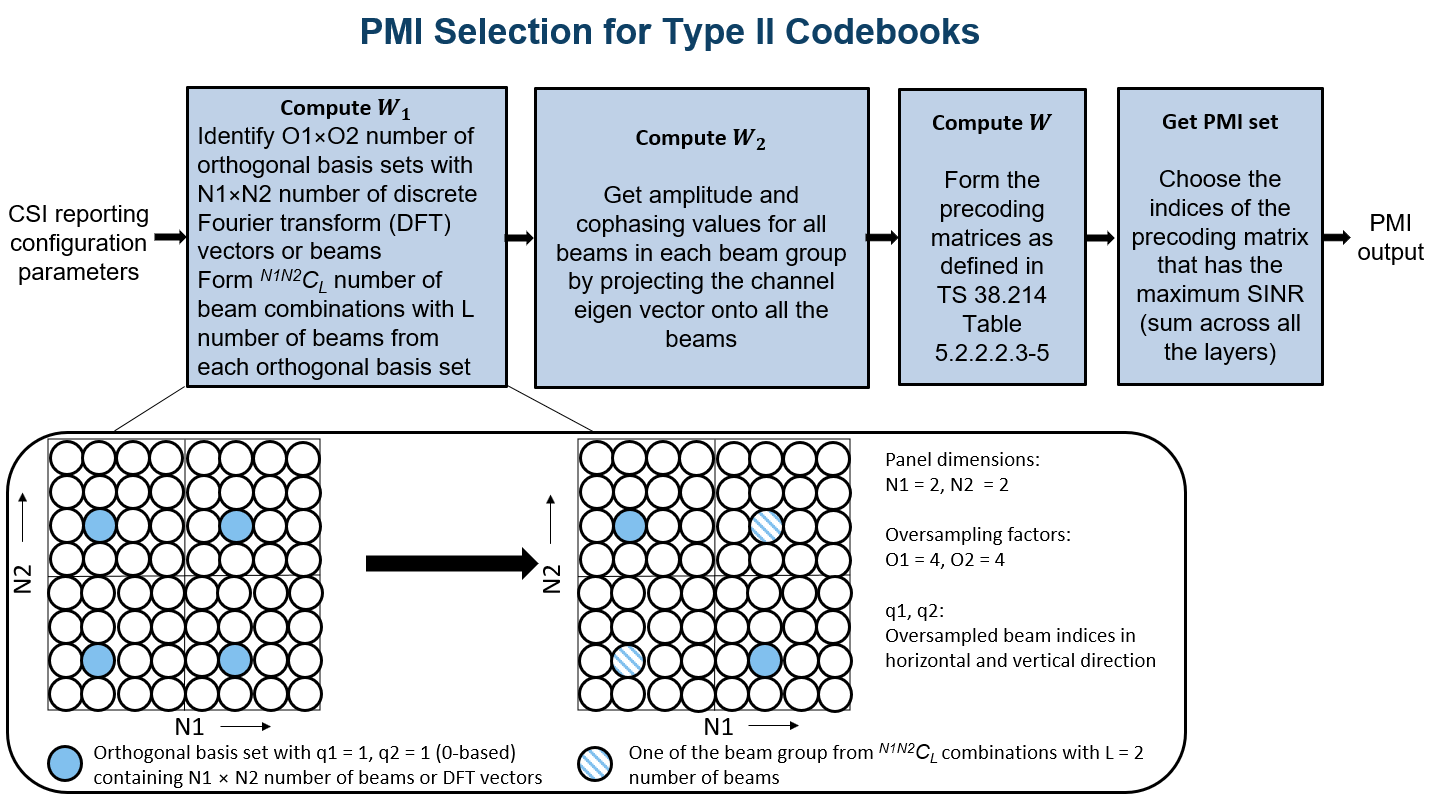#### Outline of Example

This example shows how to compute the CQI and PMI indices for a 4-by-4 MIMO scenario over a TDL channel with delay profile TDL-C, a delay spread of 300e-9 seconds, and a maximum doppler shift of 50 Hz. It compares the time-domain and frequency-domain variations of CQI values against the time-domain and frequency-domain variations of SINR values for both practical and perfect channel estimation scenarios. The example also highlights the variations of PMI values across time-domain and frequency-domain for practical and perfect channel estimation scenarios.

### Simulation Length and SNR Point

Set the length of the simulation in terms of the number of 10 ms frames. The SNR (SINR with zero interference) is defined per resource element (RE) and applies to each receive antenna.

```nFrames = 5; % Number of 10 ms frames SNRdB = 0; % SNR in dB```

### Carrier, Bandwidth Part, and CSI-RS Configuration

Create a carrier configuration object representing a 10 MHz carrier with a subcarrier spacing of 15 kHz.

```carrier = nrCarrierConfig; carrier.SubcarrierSpacing = 15; carrier.NSizeGrid = 52;```

Configure the size of the bandwidth part (BWP) and the start of the BWP relative to common resource block 0 (CRB 0).

```NStartBWP = 0; NSizeBWP = 52;```

Create a CSI-RS configuration object representing a non-zero-power CSI-RS (NZP-CSI-RS) resource set with a set of NZP-CSI-RS resources. Make sure that the NZP-CSI-RS resources that are configured in this example have the same code division multiplexing (CDM) types and the same number of CSI-RS ports, as defined in TS 38.214 Section 5.2.2.3.1.

```csirs = nrCSIRSConfig; csirs.CSIRSType = {'nzp','nzp','nzp'}; csirs.RowNumber = [4 4 4]; csirs.NumRB = 52; csirs.RBOffset = 0; csirs.CSIRSPeriod = [4 0]; csirs.SymbolLocations = {0, 0, 0}; csirs.SubcarrierLocations = {0, 4, 8}; csirs.Density = {'one','one','one'};```

#### Other Simulation-wide Parameters

Configure the number of transmit and receive antennas. The number of transmit antennas must be greater than or equal to the number of CSI-RS ports.

```nTxAnts = 4; nRxAnts = 4;```

Validate the CSI-RS configuration object.

`validateCSIRSConfig(carrier,csirs,nTxAnts);`

Configure the number of transmission layers. Number of layers from 1 to 4 corresponds to one codeword and the number of layers from 5 to 8 corresponds to two codewords. The number of transmission layers must be less than or equal to the minimum of the `nTxAnts` and `nRxAnts`.

`numLayers = 4;`

### CSI Reporting Configuration

These are the parameters required for the CQI and PMI computation and are specified as a structure with fields:

• NSizeBWP: Size of the BWP in terms of the number of physical resource blocks (PRBs)

• NStartBWP: Starting PRB index of the BWP relative to CRB 0

• PanelDimensions: The antenna panel dimensions as [N1 N2] according to TS 38.214 Table 5.2.2.2-2, as shown in the figure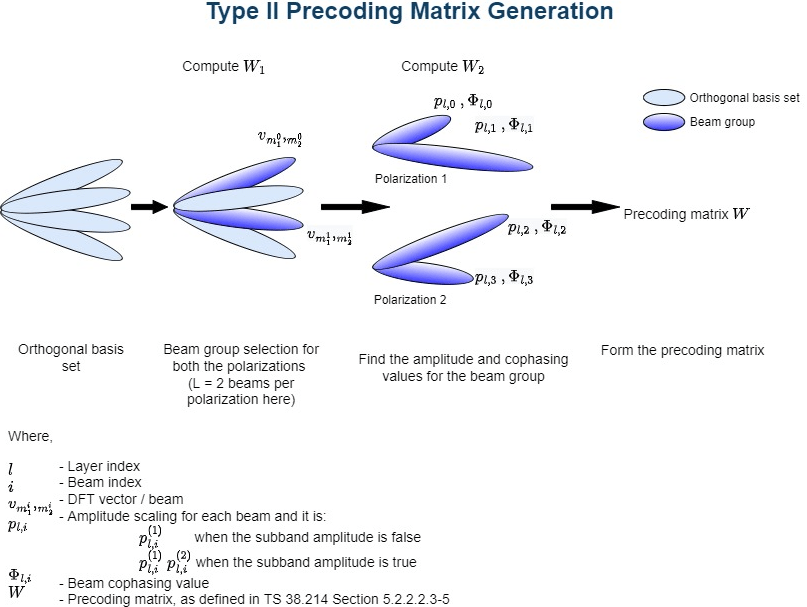• CQIMode: The mode of CQI reporting ('Subband', 'Wideband')

• PMIMode: The mode of PMI reporting ('Subband', 'Wideband')

• SubbandSize: The size of the subband, as defined in TS 38.214 Table 5.2.1.4-2 (required only when CQIMode or PMIMode is 'Subband')

• PRGSize: The precoding resource block group (PRG) size for the CQI calculation (required only when the reporting must be done for the report quantity 'cri-RI-i1-CQI' as defined in TS 38.214 Section 5.2.1.4.2)

• CodebookMode: The codebook mode (required only when the number of transmission layers is 1 or 2 and the number of CSI-RS ports is greater than 2)

• CodebookSubsetRestriction: Restriction parameter pertaining to the codebook index set ${\mathit{i}}_{1}$, that is, vlm or vbarlm restriction parameter, as defined in TS 38.214 Section 5.2.2.2.1. This field denotes the set of ${\mathit{i}}_{1}$ indices (${\mathit{i}}_{1}$ is a three element vector of the form $\left[{\mathit{i}}_{11\text{\hspace{0.17em}}},\text{\hspace{0.17em}}{\mathit{i}}_{12\text{\hspace{0.17em}}\text{\hspace{0.17em}}},\text{\hspace{0.17em}}{\mathit{i}}_{13\text{\hspace{0.17em}}\text{\hspace{0.17em}}}\right]$) that are restricted from the consideration for the PMI computation.

• i2Restriction: Restriction parameter pertaining to the codebook index ${\mathit{i}}_{2}$. This field denotes the set of ${\mathit{i}}_{2}$ indices that are restricted from the consideration for the PMI computation.

```% Configure CSI reporting configuration parameters reportConfig.NStartBWP = NStartBWP; reportConfig.NSizeBWP = NSizeBWP; reportConfig.PanelDimensions = [2 1]; reportConfig.CQIMode = 'Subband'; reportConfig.PMIMode = 'Subband'; reportConfig.SubbandSize = 4; reportConfig.PRGSize = []; reportConfig.CodebookMode = 1; reportConfig.CodebookSubsetRestriction = []; reportConfig.i2Restriction = [];```

### Propagation Channel Model Configuration

Consider a TDL channel with delay profile TDL-C, a delay spread of 300e-9 seconds, and a maximum doppler shift of 50 Hz.

```% Create channel object channel = nrTDLChannel; channel.NumTransmitAntennas = nTxAnts; channel.NumReceiveAntennas = nRxAnts; channel.DelayProfile = 'TDL-C'; channel.MaximumDopplerShift = 50; channel.DelaySpread = 300e-9;```

Set the sampling rate for the channel model by using the value returned from the `nrOFDMInfo` function.

```waveformInfo = nrOFDMInfo(carrier); channel.SampleRate = waveformInfo.SampleRate;```

Get the maximum number of delayed samples by a channel multipath component. The maximum number of delayed samples is calculated from the channel path with the largest delay and the implementation delay of the channel filter. The number of samples that correspond to the maximum channel delay is required later to flush the channel filter to obtain the received signal.

```chInfo = info(channel); maxChDelay = ceil(max(chInfo.PathDelays*channel.SampleRate)) + chInfo.ChannelFilterDelay;```

### Processing Loop

For each slot, the CSI-RS is generated, transmitted through the channel, and then processed at the receiver side to compute the CQI and PMI values. The transmit to receive end processing for each slot follows these steps:

1. Generate the resource grid: Generate the slot grid that is populated with the CSI-RS and map the slot grid from CSI-RS ports to transmit antennas.

2. Generate the waveform: OFDM modulate the generated grid by using the `nrOFDMModulate` function.

3. Model and apply a noisy channel: Pass the waveform through a TDL-C fading channel, and then add the additive white Gaussian noise (AWGN). The SNR is defined per RE and applies to each receive antenna.

4. Perform timing synchronization and OFDM demodulation: For practical synchronization, correlate the received waveform with the CSI-RS. For perfect synchronization, use the path gains and path filters of the channel are used. The synchronized signal is then OFDM demodulated with the `nrOFDMDemodulate` function.

5. Perform channel estimation: For practical channel estimation, use the CSI-RS. For perfect channel estimation, use the path gains, the path filters, and the sample times of channel snapshots.

6. Compute CQI and PMI values: Compute CQI and PMI values using practical and perfect channel estimations.

```% Initialize variables to store CQI, PMI, and subband SINR values for % practical and perfect channel estimation scenarios cqiPracticalPerSlot = []; subbandCQIPractical = []; pmiPracticalPerSlot = struct('i1',[],'i2',[]); SINRPerSubbandPerCWPractical = []; cqiPerfectPerSlot = []; subbandCQIPerfect = []; pmiPerfectPerSlot = struct('i1',[],'i2',[]); SINRPerSubbandPerCWPerfect = []; % Calculate total number of slots totSlots = nFrames*carrier.SlotsPerFrame; % Get number of CSI-RS ports csirsPorts = csirs.NumCSIRSPorts(1); % Get CDM lengths corresponding to configured CSI-RS resources cdmLengths = getCDMLengths(csirs); % Loop over all slots for slotIdx = 0:totSlots - 1 % Create carrier resource grid for one slot csirsSlotGrid = nrResourceGrid(carrier,csirsPorts); % Update slot number in carrier configuration object carrier.NSlot = slotIdx; % Generate CSI-RS indices and symbols csirsInd = nrCSIRSIndices(carrier,csirs); csirsSym = nrCSIRS(carrier,csirs); % Map CSI-RS to slot grid csirsSlotGrid(csirsInd) = csirsSym; % Map CSI-RS ports to transmit antennas wtx = eye(csirsPorts,nTxAnts); txGrid = reshape(reshape(csirsSlotGrid,[],csirsPorts)*wtx,size(csirsSlotGrid,1),size(csirsSlotGrid,2),nTxAnts); % Perform OFDM modulation to generate time-domain waveform txWaveform = nrOFDMModulate(carrier,txGrid); % Append zeros at the end of the transmitted waveform to flush channel % content. These zeros take into account any delay introduced in the % channel. The channel delay is a mix of multipath delay and % implementation delay. This value may change depending on the sampling % rate, delay profile, and delay spread. txWaveform = [txWaveform; zeros(maxChDelay,size(txWaveform,2))]; %#ok<AGROW> % Transmit waveform through channel [rxWaveform,pathGains,sampleTimes] = channel(txWaveform); % Generate and add AWGN to received waveform SNR = 10^(SNRdB/20); % Linear SNR value N0 = 1/(sqrt(2.0*nRxAnts*double(waveformInfo.Nfft))*SNR); % Noise standard deviation rng('default'); % Set RNG state for repeatability noise = N0*complex(randn(size(rxWaveform)),randn(size(rxWaveform))); rxWaveform = rxWaveform + noise; % Perform practical timing estimation offsetPractical = nrTimingEstimate(carrier,rxWaveform,csirsInd,csirsSym); if offsetPractical > maxChDelay offsetPractical = 0; end % Get path filters pathFilters = getPathFilters(channel); % Perform perfect timing estimation offsetPerfect = nrPerfectTimingEstimate(pathGains,pathFilters); % Perform time-domain offset correction for practical and % perfect timing estimation scenarios rxWaveformPractical = rxWaveform(1+offsetPractical:end,:); rxWaveformPerfect = rxWaveform(1+offsetPerfect:end,:); % Perform OFDM demodulation on previously synchronized waveforms rxGridPractical = nrOFDMDemodulate(carrier,rxWaveformPractical); rxGridPerfect = nrOFDMDemodulate(carrier,rxWaveformPerfect); % Consider only the NZP-CSI-RS symbols for channel estimation nzpCSIRSSym = csirsSym(csirsSym ~= 0); % Consider only the NZP-CSI-RS indices for channel estimation nzpCSIRSInd = csirsInd(csirsSym ~= 0); % Calculate practical channel estimate using 'AveragingWindow' as [5 1] [PracticalHest,nVarPractical] = nrChannelEstimate(carrier,rxGridPractical, ... nzpCSIRSInd,nzpCSIRSSym,'CDMLengths',cdmLengths,'AveragingWindow',[5 1]); % Perform perfect channel estimation PerfectHest = nrPerfectChannelEstimate(carrier,pathGains,pathFilters,offsetPerfect,sampleTimes); % Get perfect noise estimate value from noise realization noiseGrid = nrOFDMDemodulate(carrier,noise(1+offsetPerfect:end,:)); nVarPerfect = var(noiseGrid(:)); % Calculate CQI and PMI values using practical channel estimate [cqiPractical,pmiPractical,cqiInfoPractical] = hCQISelect(carrier,csirs, ... reportConfig,numLayers,PracticalHest,nVarPractical); % Store CQI, PMI, and subband SINR values of each slot for % practical channel estimation scenario cqiPracticalPerSlot(:,:,slotIdx+1) = cqiPractical; %#ok<SAGROW> pmiPracticalPerSlot(slotIdx+1) = pmiPractical; subbandCQIPractical(:,:,slotIdx+1) = cqiInfoPractical.SubbandCQI; %#ok<SAGROW> SINRPerSubbandPerCWPractical(:,:,slotIdx+1) = cqiInfoPractical.SINRPerSubbandPerCW; %#ok<SAGROW> % Calculate CQI and PMI values using perfect channel estimate [cqiPerfect,pmiPerfect,cqiInfoPerfect] = hCQISelect(carrier,csirs, ... reportConfig,numLayers,PerfectHest,nVarPerfect); % Store CQI, PMI, and subband SINR values of each slot for % perfect channel estimation scenario cqiPerfectPerSlot(:,:,slotIdx+1) = cqiPerfect; %#ok<SAGROW> subbandCQIPerfect(:,:,slotIdx+1) = cqiInfoPerfect.SubbandCQI; %#ok<SAGROW> pmiPerfectPerSlot(slotIdx+1) = pmiPerfect; SINRPerSubbandPerCWPerfect(:,:,slotIdx+1) = cqiInfoPerfect.SINRPerSubbandPerCW; %#ok<SAGROW> end```

### Compare CQI and PMI Values Reported in Practical and Perfect Channel Estimation Scenarios

#### Plot CQI Indices

Plot the wideband SINR values and wideband CQI values for each codeword for practical and perfect channel estimation scenarios. The plot highlights only the slots in which the CSI-RS is transmitted, or the CQI is calculated. The figure shows how the SINR and the corresponding reported CQI vary across the slots due to channel fading.

`plotWidebandCQIAndSINR(cqiPracticalPerSlot,cqiPerfectPerSlot,SINRPerSubbandPerCWPractical,SINRPerSubbandPerCWPerfect);`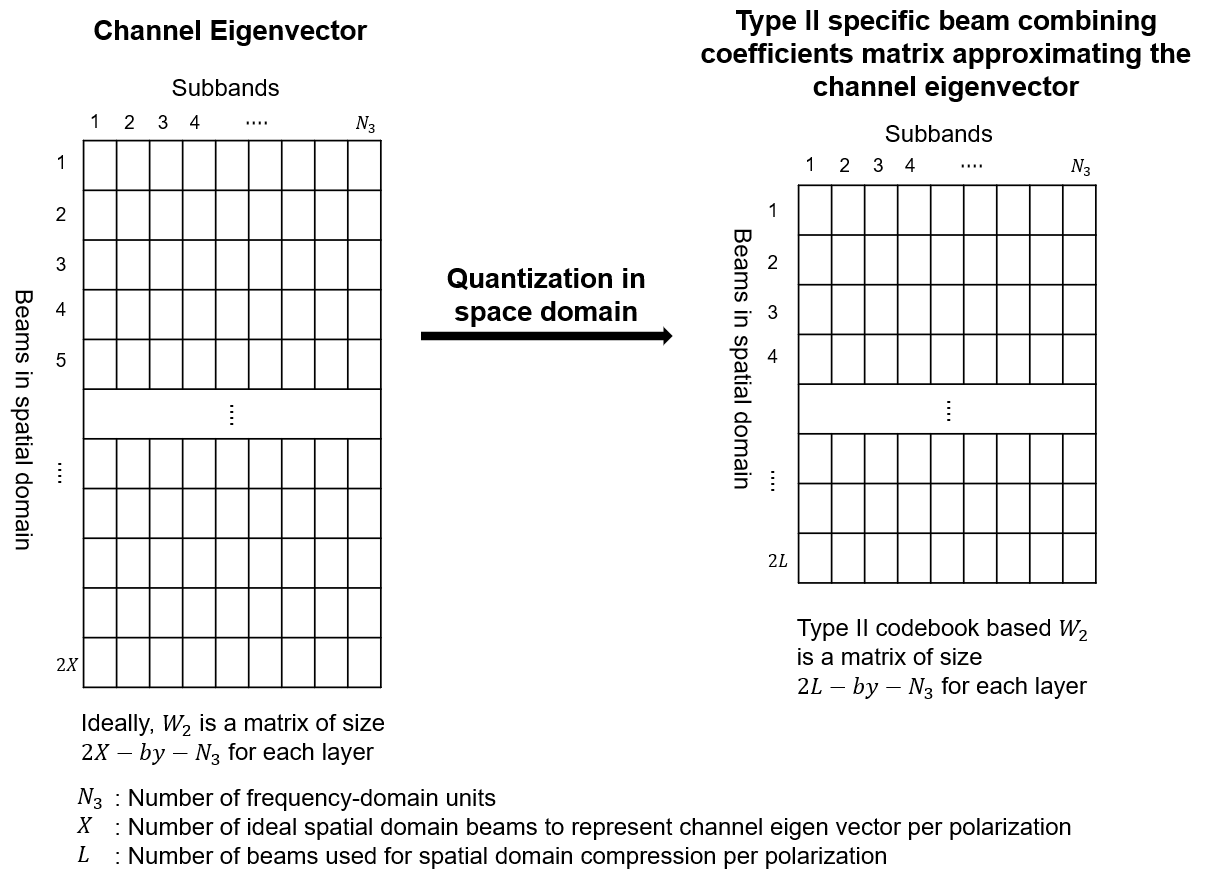Plot the subband SINR values and subband CQI values for each codeword for both practical and perfect channel estimation scenarios. The plot highlights the variations of SINR and CQI values across the subbands for a specified slot number (1-based) if CQI is reported in that slot. Otherwise, no plot is generated because the CQI is not reported.

The `plotSubbandCQIAndSINR` function plots the CQI values across all subbands only when the CQI mode is configured as 'Subband'.

```% Provide slot number for which subband CQI values and PMI i2 indices must be plotted slotNum = 1; % Consider slot number as 1 (1-based) here, because CSI (CQI and PMI) is % reported in that slot for the configured CSI-RS resource(s) plotSubbandCQIAndSINR(subbandCQIPractical,subbandCQIPerfect,SINRPerSubbandPerCWPractical,SINRPerSubbandPerCWPerfect,slotNum);```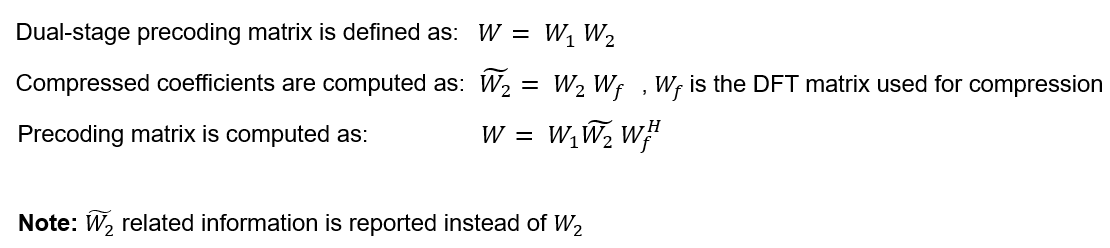#### Plot PMI Indices

This section shows the plots of PMI indices (${\mathit{i}}_{1}:\left[{\mathit{i}}_{1,1},{\mathit{i}}_{1,2},{\mathit{i}}_{1,3}\right]$ and ${\mathit{i}}_{2}$). The plot highlights only the slots in which the CSI-RS is transmitted, or the PMI is calculated. The plot highlights the variations in the ${\mathit{i}}_{1}$ indices across the slots due to channel fading. The plot also highlights the variation in ${\mathit{i}}_{2}$ indices across:

• The slots, if the PMIMode is configured as 'Wideband'

• The subbands in the specified slot, if the PMIMode is configured as 'Subband'

`plotPMI(pmiPracticalPerSlot,pmiPerfectPerSlot,slotNum);`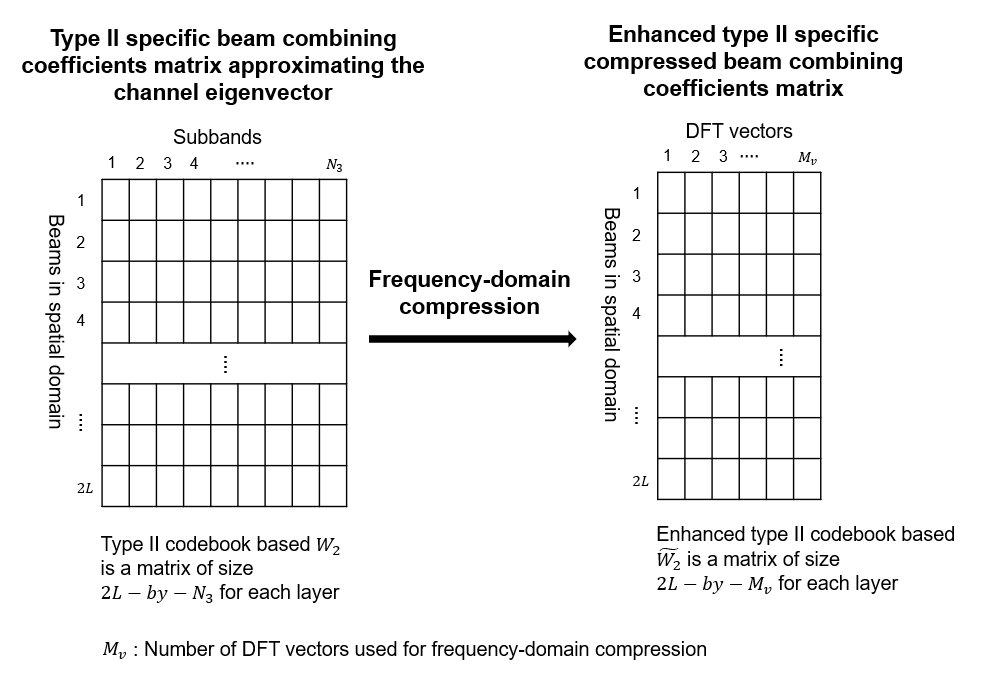### Summary and Further Exploration

The example shows how to compute downlink CSI parameters such as the CQI and PMI from type-1 single panel codebooks for a MIMO scenario with a TDL channel.

You can modify carrier, channel, CSI-RS resource configurations, and CSI reporting configuration parameters (such as the mode of CQI and PMI reporting, and the subband size) and observe the variations in the computed CQI and PMI values across the time (slots) and frequency (subbands).

### References

1. 3GPP TS 38.214. "NR; Physical layer procedures for data." 3rd Generation Partnership Project; Technical Specification Group Radio Access Network.

### Local Functions

The example uses these local functions to validate the CSI-RS configuration object, and to plot the computed CQI and PMI values

```function validateCSIRSConfig(carrier,csirs,nTxAnts) % validateCSIRSPorts(CARRIER,CSIRS,NTXANTS) validates the CSI-RS % configuration, given the carrier specific configuration object CARRIER, % CSI-RS configuration object CSIRS, and the number of transmit antennas % NTXANTS. % Validate the number of CSI-RS ports if ~isscalar(unique(csirs.NumCSIRSPorts)) error('nr5g:InvalidCSIRSPorts',... 'All the CSI-RS resources must be configured to have the same number of CSI-RS ports.'); end % Validate the CDM lengths if ~iscell(csirs.CDMType) cdmType = {csirs.CDMType}; else cdmType = csirs.CDMType; end if (~all(strcmpi(cdmType,cdmType{1}))) error('nr5g:InvalidCSIRSCDMTypes',... 'All the CSI-RS resources must be configured to have the same CDM lengths.'); end if nTxAnts < csirs.NumCSIRSPorts(1) error('nr5g:InvalidNumTxAnts',['Number of transmit antennas (' num2str(nTxAnts)... ') must be greater than or equal to the number of CSI-RS ports (' num2str(csirs.NumCSIRSPorts(1)) ').']); end % Check for the overlap between the CSI-RS indices csirsInd = nrCSIRSIndices(carrier,csirs,"OutputResourceFormat",'cell'); numRes = numel(csirsInd); csirsIndAll = cell(1,numRes); ratioVal = csirs.NumCSIRSPorts(1)/prod(getCDMLengths(csirs)); for resIdx = 1:numRes if ~isempty(csirsInd{resIdx}) grid = nrResourceGrid(carrier,csirs.NumCSIRSPorts(1)); [~,tempInd] = nrExtractResources(csirsInd{resIdx},grid); if numel(tempInd)/numel(csirsInd{resIdx}) ~= ratioVal error('nr5g:OverlappedCSIRSREsSingleResource',['CSI-RS indices of resource '... num2str(resIdx) ' must be unique. Try changing the symbol or subcarrier locations.']); end csirsIndAll{resIdx} = tempInd(:); for idx = 1:resIdx-1 overlappedInd = ismember(csirsIndAll{idx},csirsIndAll{resIdx}); if any(overlappedInd) error('nr5g:OverlappedCSIRSREsMultipleResources',['The resource elements of the '... 'configured CSI-RS resources must not overlap. Try changing the symbol or '... 'subcarrier locations of CSI-RS resource ' num2str(idx) ' and resource ' num2str(resIdx) '.']); end end end end end function cdmLengths = getCDMLengths(csirs) % CDMLENGTHS = getCDMLengths(CSIRS) returns the CDM lengths given the % CSI-RS configuration object CSIRS. CDMType = csirs.CDMType; if ~iscell(csirs.CDMType) CDMType = {csirs.CDMType}; end CDMTypeOpts = {'noCDM','fd-CDM2','CDM4','CDM8'}; CDMLengthOpts = {[1 1],[2 1],[2 2],[2 4]}; cdmLengths = CDMLengthOpts{strcmpi(CDMTypeOpts,CDMType{1})}; end function plotWidebandCQIAndSINR(cqiPracticalPerSlot,cqiPerfectPerSlot,SINRPerSubbandPerCWPractical,SINRPerSubbandPerCWPerfect) % plotWidebandCQIAndSINR(CQIPRACTICALPERSLOT,CQIPERFECTPERSLOT,SINRPERSUBBANDPERCWPRACTICAL,SINRPERSUBBANDPERCWPERFECT) % plots the wideband SINR and wideband CQI values for each codeword % across all active slots (in which the CQI is reported as other % than NaN) for practical and perfect channel estimation cases. numCW = size(cqiPracticalPerSlot,2); % Plot wideband CQI values for cwIdx = 1:numCW cqiPracticalPerCW = permute(cqiPracticalPerSlot(1,cwIdx,:),[1 3 2]); cqiPerfectPerCW = permute(cqiPerfectPerSlot(1,cwIdx,:),[1 3 2]); SINRPerCWPractical = permute(SINRPerSubbandPerCWPractical(1,cwIdx,:),[1 3 2]); SINRPerCWPerfect = permute(SINRPerSubbandPerCWPerfect(1,cwIdx,:),[1 3 2]); % Get logical array to represent active slots in which CQI is % reported activeSlotsLogAry = ~isnan(cqiPracticalPerCW); % Get slot numbers (0-based) in which CQI is reported activeSlotNum = find(activeSlotsLogAry)-1; % Extract wideband CQI indices for slots where CQI is reported % as other than NaN cqiPracticalPerCWActiveSlots = cqiPracticalPerCW(activeSlotsLogAry); cqiPerfectPerCWActiveSlots = cqiPerfectPerCW(activeSlotsLogAry); widebandSINRPractical = 10*log10(SINRPerCWPractical(1,activeSlotsLogAry)); widebandSINRPerfect = 10*log10(SINRPerCWPerfect(1,activeSlotsLogAry)); xTickLables = num2cell(activeSlotNum(:)); if isempty(cqiPracticalPerCWActiveSlots) disp('No CQI data to plot, because all CQI values are NaNs.'); return; end figure(); subplot(211) plot(widebandSINRPerfect,'r^-') hold on; plot(widebandSINRPractical,'b^-') title(['Estimated Wideband SINR Values for Codeword ' num2str(cwIdx)]); xlabel('Slots'); ylabel('Wideband SINR Values in dB'); xticks(1:numel(cqiPracticalPerCWActiveSlots)); xticklabels(xTickLables); [lowerBound,upperBound] = bounds([widebandSINRPractical(:);widebandSINRPerfect(:)]); ylim([lowerBound-1 upperBound+3.5]); legend({'Perfect channel est.','Practical channel est.'}); subplot(212) plot(cqiPerfectPerCWActiveSlots,'r-o'); hold on; plot(cqiPracticalPerCWActiveSlots,'b-*'); xlabel('Slots'); xticks(1:numel(cqiPracticalPerCWActiveSlots)); xticklabels(xTickLables); ylabel('Wideband CQI'); [lowerBound,upperBound] = bounds([cqiPracticalPerCWActiveSlots(:);cqiPerfectPerCWActiveSlots(:)]); ylim([lowerBound-1 upperBound+3.5]); title(['Wideband CQI Values for Codeword ' num2str(cwIdx)]); legend({'Perfect channel est.','Practical channel est.'}); end end function plotSubbandCQIAndSINR(subbandCQIPractical,subbandCQIPerfect,SINRPerCWPractical,SINRPerCWPerfect,nslot) % plotSUBBANDCQIAndSINR(SUBBANDCQIPRACTICAL,SUBBANDCQIPERFECT,SINRPERCWPRACTICAL,SINRPERCWPERFECT,NSLOT) % plots the SINR and CQI values for each codeword across all subbands % for practical and perfect channel estimation cases for the given slot % NSLOT. The function does not plot the values if CQIMode is % 'Wideband' or if the CQI and SINR values are all NaNs. numCW = size(subbandCQIPractical,2); numSubbands = size(subbandCQIPractical,1); if numSubbands > 1 Mode = 'Subband'; else Mode = 'Wideband'; end % Plot subband CQI values if strcmpi(Mode,'subband') for cwIdx = 1:numCW subbandCQIPerCWPractical = subbandCQIPractical(2:end,cwIdx,nslot); subbandCQIPerCWPerfect = subbandCQIPerfect(2:end,cwIdx,nslot); subbandSINRPerCWPractical = 10*log10(SINRPerCWPractical(2:end,cwIdx,nslot)); subbandSINRPerCWPerfect = 10*log10(SINRPerCWPerfect(2:end,cwIdx,nslot)); xTickLables = num2cell(1:numel(subbandCQIPerfect(2:end,cwIdx,1))); if all(isnan(subbandCQIPerCWPractical)) disp(['For the specified slot (' num2str(nslot) '), CQI values are not reported. Please choose another slot number.']) else figure(); subplot(211) plot(subbandSINRPerCWPerfect,'r^-') hold on; plot(subbandSINRPerCWPractical,'b^-') title(['Estimated Subband SINR Values for Codeword ' num2str(cwIdx)]); xlabel('Subbands'); ylabel('Subband SINR Values in dB'); xticks(1:numSubbands); xticklabels(xTickLables); xlim([0 numSubbands+1]); [lowerBound,upperBound] = bounds([subbandSINRPerCWPerfect;subbandSINRPerCWPractical]); ylim([lowerBound-1 upperBound+3.5]); legend({'Perfect channel est.','Practical channel est.'}); subplot(212) plot(subbandCQIPerCWPerfect,'r-o'); hold on; plot(subbandCQIPerCWPractical,'b-*'); title(['Subband CQI Values for Codeword ' num2str(cwIdx)]); xlabel('Subbands'); ylabel('Subbands CQI'); xticks(1:numSubbands); xticklabels(xTickLables); xlim([0 numSubbands+1]); [lowerBound,upperBound] = bounds([subbandCQIPerCWPerfect(:,1);subbandCQIPerCWPractical(:,1)]); ylim([lowerBound-1 upperBound+3.5]); legend({'Perfect channel est.','Practical channel est.'}); end end end end function plotPMI(pmiPracticalPerSlot,pmiPerfectPerSlot,nslot) % plotPMI(PMIPRACTICALPERSLOT,PMIPERFECTPERSLOT,NSLOT) plots the PMI i1 % indices across all active slots for practical and perfect % channel estimation scenarios. The function also plots the i2 indices of % practical and perfect channel estimation scenarios across all % active slots, when the PMI mode is 'Wideband' or plots i2 indices % across all subbands for the specified slot number NSLOT when the % PMI mode is 'Subband'. % Extract i1 values for slots where PMI is reported as other % than NaN i1PerfectVals = reshape([pmiPerfectPerSlot(:).i1],3,[])'; i1PracticalVals = reshape([pmiPracticalPerSlot(:).i1],3,[])'; % Get logical array to represent active slots in which PMI is % reported activeSlotsLogAry = ~isnan(i1PerfectVals(:,1)); % Get slot numbers (0-based) in which PMI is reported activeSlotNum = find(activeSlotsLogAry)-1; % Get number of active slots numActiveSlots = numel(activeSlotNum); % Extract wideband PMI indices for slots where PMI is reported % as other than NaN i1PerfectValsActiveSlots = i1PerfectVals(activeSlotsLogAry,:); i1PracticalValsActiveSlots = i1PracticalVals(activeSlotsLogAry,:); if isempty(i1PerfectValsActiveSlots) disp('No PMI data to plot, because all PMI values are NaNs.'); return; end % Get i11 indices i11PerfectVals = i1PerfectValsActiveSlots(:,1); i11PracticalVals = i1PracticalValsActiveSlots(:,1); % Plot i11 values figure; subplot(411) plot(i11PerfectVals,'r-o'); hold on; plot(i11PracticalVals,'b-*'); xlabel('Slots') ylabel('i11 Indices'); xticks(1:numActiveSlots); xTickLables = num2cell(activeSlotNum(:)); xticklabels(xTickLables); [lowerBound,upperBound] = bounds([i11PerfectVals; i11PracticalVals]); ylim([lowerBound-2 upperBound+2]); title('PMI: i11 Indices') legend({'Perfect channel est.','Practical channel est.'}); % Get i12 indices i12PerfectVals = i1PerfectValsActiveSlots(:,2); i12PracticalVals = i1PracticalValsActiveSlots(:,2); % Plot i12 values subplot(412) plot(i12PerfectVals,'r-o'); hold on; plot(i12PracticalVals,'b-*'); xlabel('Slots') ylabel('i12 Indices'); xticks(1:numActiveSlots); xticklabels(xTickLables); [lowerBound,upperBound] = bounds([i12PerfectVals; i12PracticalVals]); ylim([lowerBound-2 upperBound+2]); title('PMI: i12 Indices') legend({'Perfect channel est.','Practical channel est.'}); % Get i13 indices i13PerfectVals = i1PerfectValsActiveSlots(:,3); i13PracticalVals = i1PracticalValsActiveSlots(:,3); % Plot i13 values subplot(413) plot(i13PerfectVals,'r-o'); hold on; plot(i13PracticalVals,'b-*'); xlabel('Slots') ylabel('i13 Indices'); xticks(1:numActiveSlots); xticklabels(xTickLables); [lowerBound,upperBound] = bounds([i13PerfectVals; i13PracticalVals]); ylim([lowerBound-2 upperBound+2]); title('PMI: i13 Indices') legend({'Perfect channel est.','Practical channel est.'}); % Extract i2 values numSlots = numel(pmiPracticalPerSlot); i2PerfectVals = reshape([pmiPerfectPerSlot(:).i2],[],numSlots)'; % Of size NumSlots-by-NumSubbands i2PracticalVals = reshape([pmiPracticalPerSlot(:).i2],[],numSlots)'; % Of size NumSlots-by-NumSubbands % Extract i2 values for all active slots where PMI is reported as other than % NaN i2PerfectValsActiveSlots = i2PerfectVals(activeSlotsLogAry,:); i2PracticalValsActiveSlots = i2PracticalVals(activeSlotsLogAry,:); numSubbands = size(i2PracticalVals,2); if numSubbands > 1 mode = 'Subband'; else mode = 'Wideband'; end % Plot i2 values if strcmpi(mode,'Wideband') subplot(414); plot(i2PerfectValsActiveSlots,'r-o'); hold on; plot(i2PracticalValsActiveSlots,'b-*'); title('PMI: i2 Indices') xlabel('Slots') ylabel('i2 Indices'); xticks(1:numActiveSlots); xticklabels(xTickLables); [lowerBound,upperBound] = bounds([i2PerfectValsActiveSlots; i2PracticalValsActiveSlots]); ylim([lowerBound-2 upperBound+2]); legend({'Perfect channel est.','Practical channel est.'}); else if ~all(isnan(i2PerfectVals(nslot,:))) subplot(414); plot(i2PerfectVals(nslot,:),'r-o'); hold on; plot(i2PracticalVals(nslot,:),'b-*'); title(['PMI: i2 Indices for All Subbands in Slot ' num2str(nslot)]); xlabel('Subbands') ylabel('i2 Indices'); xticks(1:numSubbands); xticklabels(num2cell(1:numSubbands)); [lowerBound,upperBound] = bounds([i2PerfectVals(nslot,:) i2PracticalVals(nslot,:)]); yticks(lowerBound:upperBound); yticklabels(num2cell(lowerBound:upperBound)); xlim([0 size(i2PerfectVals,2)+1]) ylim([lowerBound-2 upperBound+2]); legend({'Perfect channel est.','Practical channel est.'}); else disp(['For the specified slot (' num2str(nslot) '), PMI i2 indices are not reported. Please choose another slot number.']) end end end```

Download ebook